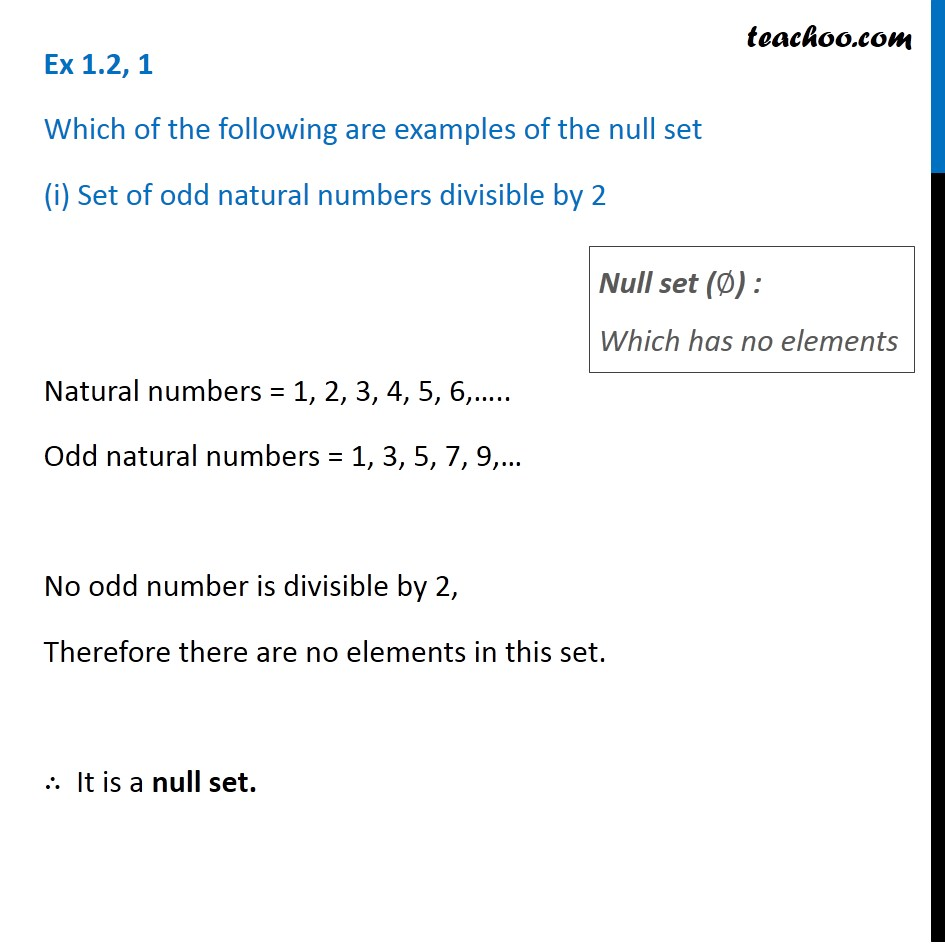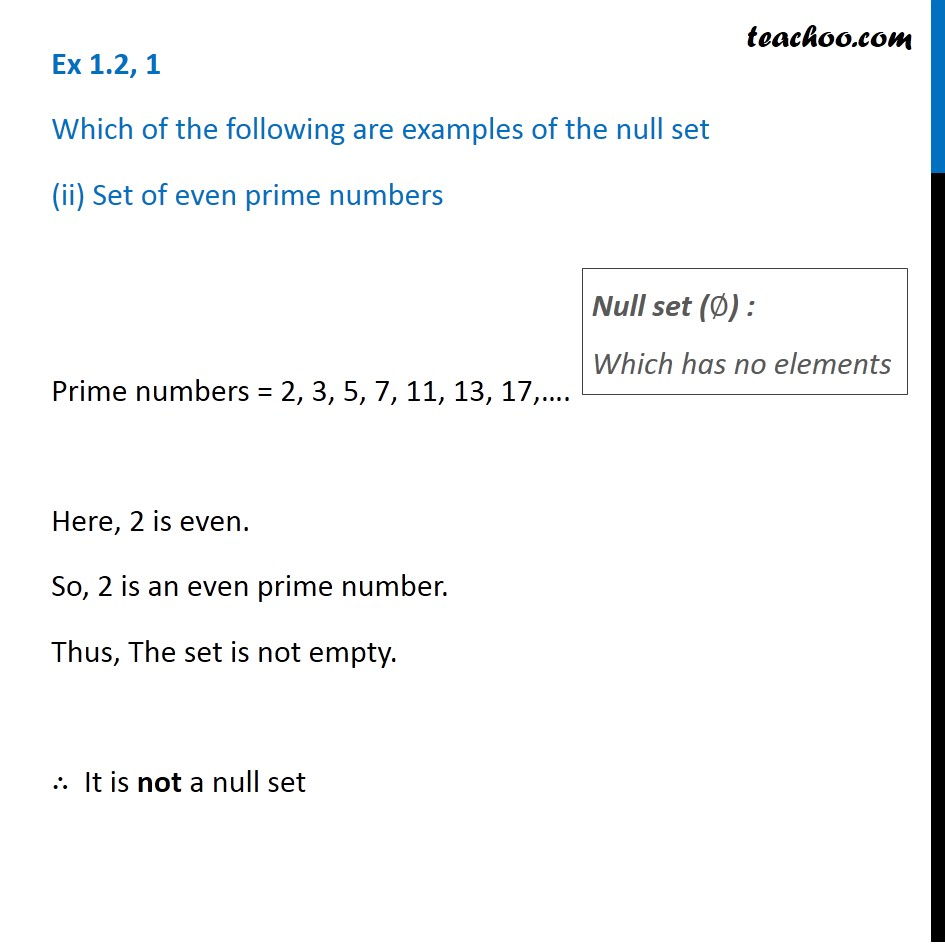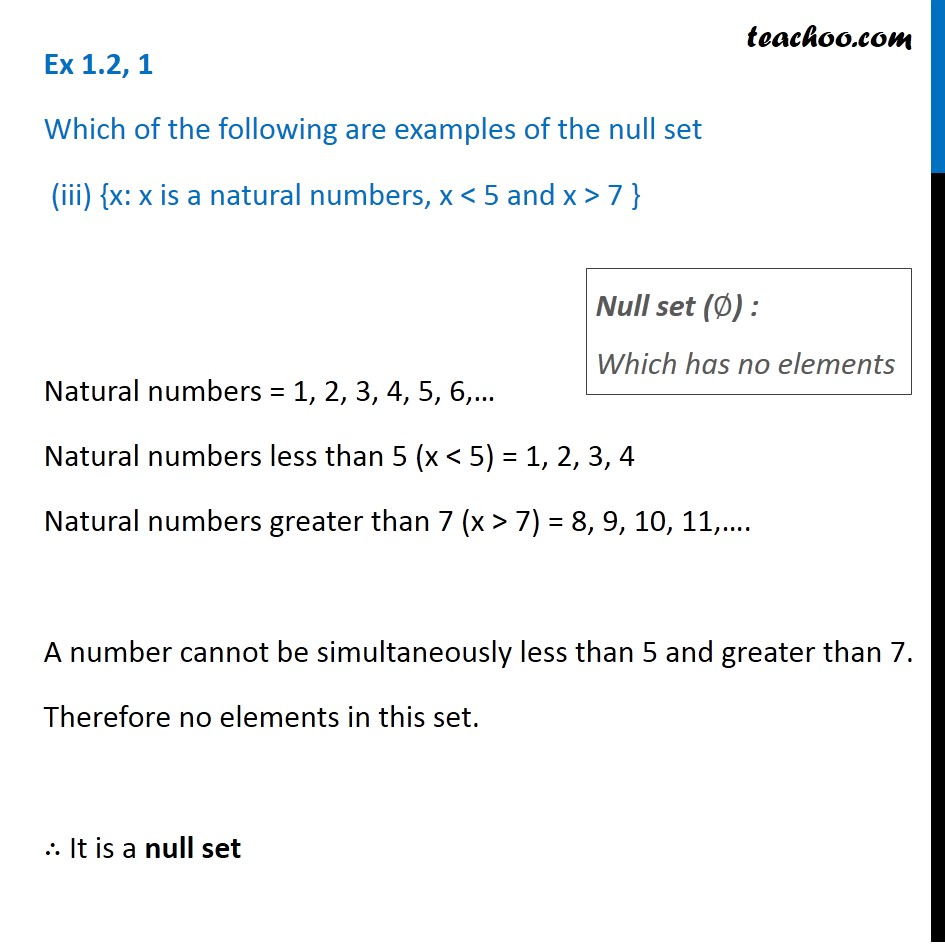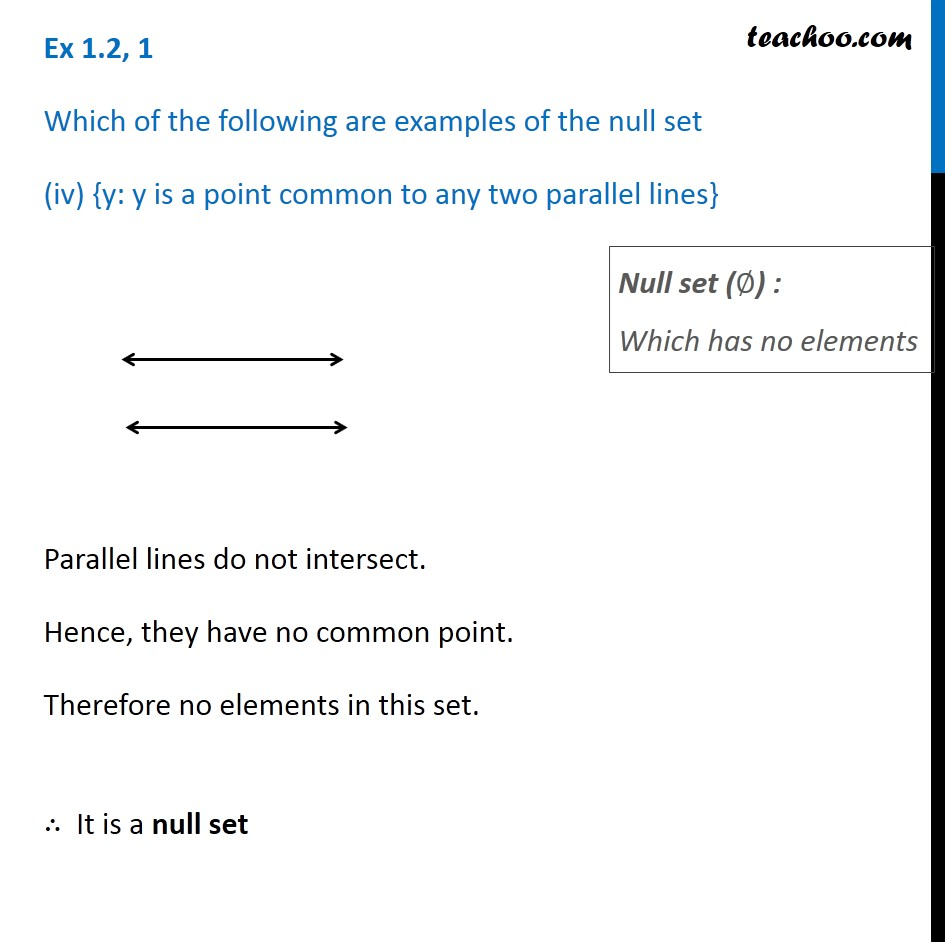1. Chapter 1 Class 11 Sets
2. Serial order wise
3. Ex 1.2

Transcript

Ex 1.2, 1 Which of the following are examples of the null set (i) Set of odd natural numbers divisible by 2 Natural numbers = 1, 2, 3, 4, 5, 6,….. Odd natural numbers = 1, 3, 5, 7, 9,… No odd number is divisible by 2, Therefore there are no elements in this set. ∴ It is a null set. Null set (∅) : Which has no elements Ex 1.2, 1 Which of the following are examples of the null set (ii) Set of even prime numbers Prime numbers = 2, 3, 5, 7, 11, 13, 17,…. Here, 2 is even. So, 2 is an even prime number. Thus, The set is not empty. ∴ It is not a null set Null set (∅) : Which has no elements Ex 1.2, 1 Which of the following are examples of the null set (iii) {x: x is a natural numbers, x < 5 and x > 7 } Natural numbers = 1, 2, 3, 4, 5, 6,… Natural numbers less than 5 (x < 5) = 1, 2, 3, 4 Natural numbers greater than 7 (x > 7) = 8, 9, 10, 11,…. A number cannot be simultaneously less than 5 and greater than 7. Therefore no elements in this set. ∴ It is a null set Null set (∅) : Which has no elements Ex 1.2, 1 Which of the following are examples of the null set (iv) {y: y is a point common to any two parallel lines} Parallel lines do not intersect. Hence, they have no common point. Therefore no elements in this set. ∴ It is a null set Null set (∅) : Which has no elements

Ex 1.2

Chapter 1 Class 11 Sets
Serial order wise